# Reliable And Efficient Compact Models For Scalable MTJ Simulation

A way to emulate the behavior of MRAMs switching at significant statistical events.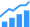By Fernando García Redondo, Pranay Prabhat, and Mudit Bhargava

Since its discovery in 1975, Tunnel-Magneto-Resistance (TMR) has been actively investigated. From the 2000s, advances in process technologies have made the miniaturization of Magnetic Random Access Memories (MRAMs) based on TMR devices possible, together with integration into traditional CMOS processes.

## Introducing magnetic random access memory technologies

Embedded Flash memory technology is limited by scaling difficulties in Flash below 28nm CMOS processes. The discovery, and later the industrial manufacturing of Spin-Transfer-Torque (STT) MRAMs, brought enough endurance, retention, scalability, and low power consumption. This positioned MRAM as the replacement of Flash as the near-future dominant Non-Volatile Memory (NVM) technology. The integration of Magnetic Tunnel Junctions (MTJs) as back-end devices in standard CMOS processes with the need of just a few extra masks ensures that MRAM is both technically and economically feasible.

As depicted in figure 1, the basic MTJ structure is made up of two ferromagnetic materials insulated by a (traditionally) oxide layer. The atomic spins in each layer constitute the layer magnetization. The pinned layer’s magnetization (mp) is fixed, but the free layer’s magnetization (m) can be altered. The resistivity of the cell is determined by the magnetization direction of the two layers. The resistance between the two terminals is minimal when both Free and Pinned layers’ (FL and PL respectively) magnetizations are parallel, (P State) and maximum when anti-parallel (AP State).

In STT MRAMs, the writing current flowing through the device produces a magnetic torque momentum over the FL magnetization, flipping it if the current is large enough. In figure 1 we described how the direction of the magnetization, pointing towards z=+/-1 in a Cartesian coordinate system defines the binary “0” and “1” states. Figure 2 describes how the magnetization vector evolves through time, from z ≈ +1, to z ≈ -1, writing a different value in the MRAM cell.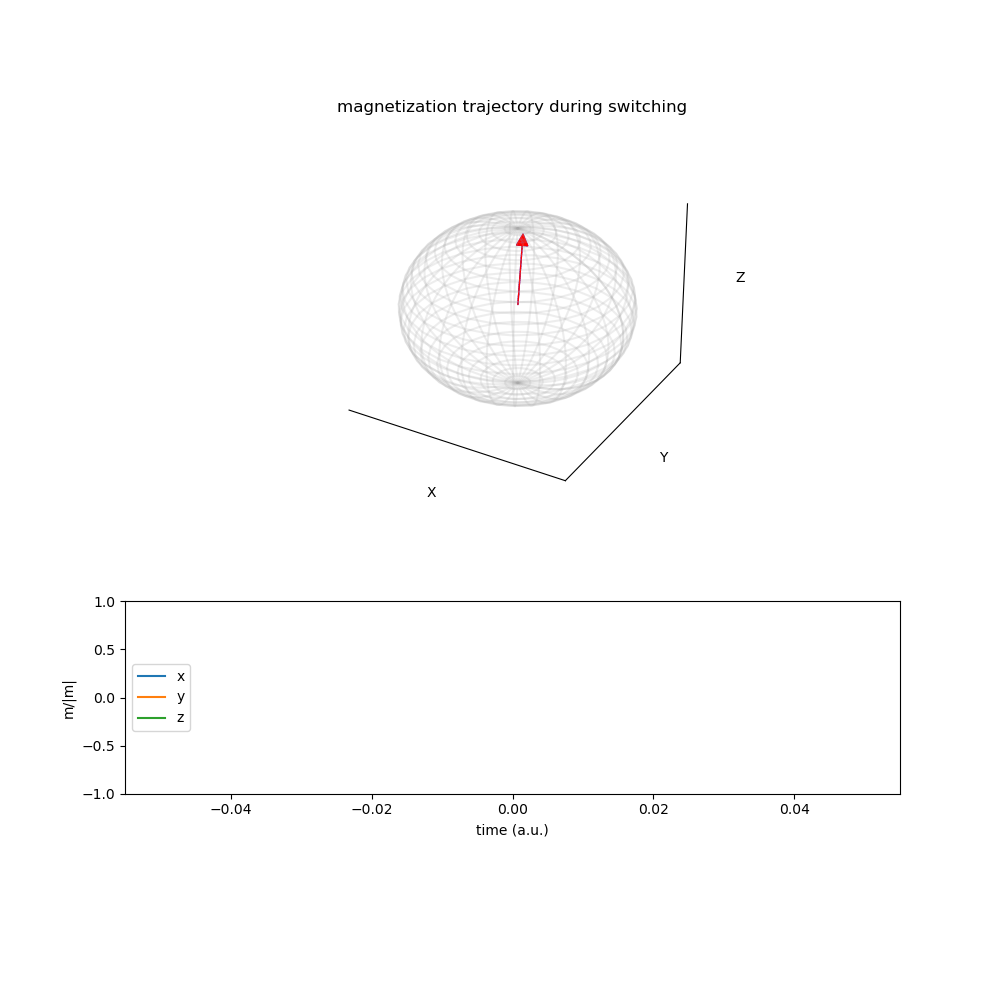Fig. 2: MRAM magnetization during switching.

The bottom graph of figure 2 describes the x, y, z components magnetization, which we will use throughout this article. The temporal evolution of the MTJ magnetization m as a monodomain nanomagnet, influenced by external and anisotropy fields, thermal noise and STT, is described by the stochastic Landau-Lifshitz-Gilbert- Slonczewsky (s-LLGS) differential equation [OOMMF].

In the equation shown in figure 3, the effective field, Heff, is determined by:

• The external field
• The anisotropy field (uniaxial and demagnetization)
• The voltage-controlled anisotropy field
• The random thermal field (H_th).

Here, γ’ refers to the gyromagnetic ratio and β ε are the magnitudes defining the transfer torque component [OOMMF]. The STT spin term is defined by the MRAM characteristics, and the current applied between the two cell terminals. The accurate computation of the magnetization, especially in the presence of the thermal random field, becomes complex and computationally costly. This means that the design of MRAM based circuits require efficient models and tools.

We present an open source framework for the simulation, characterization, and analysis of MRAM stochasticity. We also share a compact model and framework for the efficient and scalable simulation of circuits with MRAMs. We provide Verilog-A and Python compact models, able to emulate the behavior of MRAMs switching at significant statistical events. To calibrate the models for stochastic-based events, we implemented and analyzed two Fokker-Plank Equation solvers (numerical FVM and analytical). We presented an optimization module that orchestrates the efficient computation of MRAM statistics and parameter regression.

In a two-part series, related to the works “A Compact Model for Scalable MTJ Simulation“, presented at SMACD 2021, and “A Fokker-Planck Solver to Model MTJ Stochasticity“, presented at ESSDERC 2021, we share our answers to the following two problems:

• How can we reliably and efficiently simulate circuits with MRAM compact models, including stochasticity?
• How can we efficiently analyze stochasticity?

In part 1, we are diving deeper into the methodology, which is what we are most interested in as circuit designers.

## An efficient stochasticity-aware MTJ compact model for the simulation of large MRAM-based circuits

STT-MRAM circuit design needs the complex device dynamics to be incorporated into the standard SPICE-like solvers. Doing this efficiently is not an easy task. The s-LLGS system resolution, even when the stochasticity is not under consideration, can easily lead to non-convergence and error issues. We follow the work initiated by S. Ament, analyzing the integration methods and solver problems most encountered when solving s-LLGS systems, focusing on the SPICE-like circuit simulators approach.

We explore different methods to emulate the effects caused by the intrinsic stochastic nature of MRAM cells. This aims to provide circuit designers with calibrated compact models accurate enough to account for the effects caused by random fields, yet maintaining efficiency and scalability enough that they can be integrated in product-grade large circuits.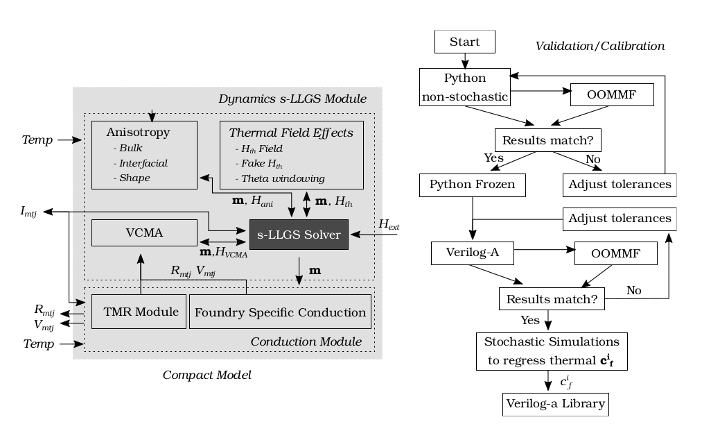Fig. 4: Proposed compact model and framework methodology.

Figure 4 describes the implemented compact model and model analysis/calibration procedure and validation against OOMMF. First, given a set of MRAM parameters, initial non-stochastic simulations are compared against OOMMF simulation results. The tolerances are adjusted until the results match. At this point, the model is frozen and exported to Verilog-A. The subsequent simulation validates the tolerances needed for the required accuracy. Finally, the coefficients of the thermal noise emulation mechanism explained in the following are regressed and the Verilog-A model library finalized.

The model is composed of two modules: Conduction and Dynamics. The Conduction scheme describing the instantaneous MTJ resistance is dependent on the foundry engineered stack. Our modular approach allows foundry-specific conduction mechanisms to complement the basic TMR scheme. The Dynamics module describes the temporal evolution of the MTJ magnetization m.

The compact model has been implemented in Python and Verilog-A. Python model supports traditional Ordinary Differential Equations (ODE) and SDE solvers, for the simulation of H_th as a pure Wiener process [S. AmentP. Horley]. The parallel Python engine enables MC and statistical studies. The Verilog-A implementation uses native integration schemes with parameterizable integration tolerances. Figure 5 describes the behavior of our Verilog-A model, and validates it against OOMMF.

As circuit designers, we are interested in the ability to analyze the most significant statistical events related to the MRAM stochastic behavior. This includes the mean switching behavior, or the switching characteristics at a given write error rate (WER 1E-6, WER 1E-8, … WER_i). Unfortunately, and as depicted in figure 6 the number of random walks required to hit a small error rate is simply too costly to run.

We provide a solution for efficient simulation of the effects caused by the stochastic H_th field, enabling the analysis of how a given circuit instantiating that MRAM device would behave statistically, with negligible simulation performance degradation. Our compact model adds a fictitious term H_fth with the purpose of emulating the H_th contribution that generates θ_0 (θ_0_i for WER_i). Thanks to this, we can efficiently extract the mean/WER_i behaviors and generate the corresponding and calibrated Verilog-A models, ready to be efficiently simulated.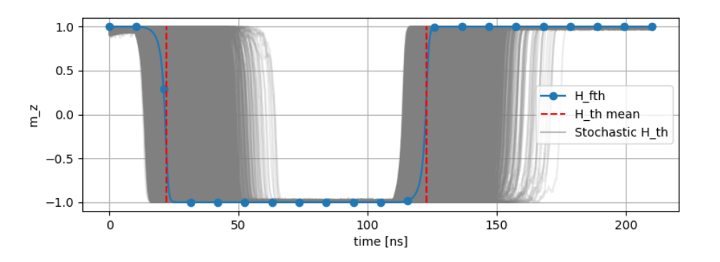Fig. 6: Stochastic SDE simulations requiring high computational resources and proposed H_fth simulation, matching the mean stochastic behavior of the cell.

## 1-Mb MRAM Macro benchmark and conclusions

To validate scalability on a commercial product, the model is instantiated into the 64 × 4 memory top block of the extracted netlist from a 1-Mb 28 nm MRAM macro [E. M. Boujamma], and simulated with macro-specific tolerance settings. The emulated magnetic term enables the previously impossible capability of simulating successive writes with identical transition times representing an MTJ with a given WER.

Figure 7 describes a writing operation 10µs after power-on sequence. We combine the s-LLGS OOMMF validated dynamics with foundry-given thermal/voltage conductance dependence, providing the accurate resistance response over time. Compared to using fixed resistors, there is a simulation overhead of 3.1× CPU time and 1.5× RAM usage. In return, circuit designers can observe accurate transient switching behavior and read disturbs.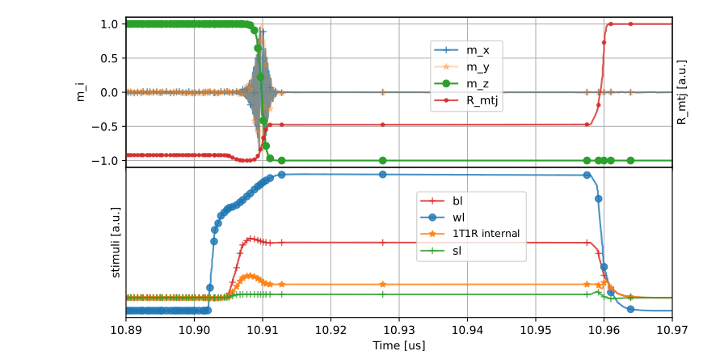Fig. 7: Magnetization, BL, WL, SL and resistance of a cell written within a 1Mb Macro.

## But the MRAM story continues…

So far, we have walked through the adventure of designing an efficient compact model that enables circuit designers to design and simulate MRAM-based circuits, taking into account stochasticity.

However, the statistical analysis of an MRAM technology is unfeasible using just s-LLGS based systems. The statistical characterization would require millions of stochastic LLGS walks, involving huge computational resources that would make the validation of a large circuit simply impossible.

In the second part, we will address this issue, and present a solution to this important problem.

The following frameworks have been presented:

• A Compact Model for Scalable MTJ Simulation, IEEE International Conference on Synthesis, Modeling, Analysis and Simulation Methods and Applications to Circuit Design, SMACD 2021.
• A Fokker-Planck Solver to Model MTJ Stochasticity European Solid-State Device Research Conference, ESSDERC 2021.

The simulation/characterization framework is available on GitHub. Read the full paper “A Compact Model for Scalable MTJ Simulation.”

Fernando García Redondo is a senior research engineer at Arm.

Pranay Prabhat is a lead for IoT circuits research at Arm.

Mudit Bhargava is a staff research engineer at Arm.

The authors would like to thank Cyrille Dray and Milos Milosavljevic for their helpful discussions.